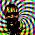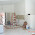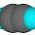## Thursday, September 1, 2011

### Fun with Filip

The Filipelli dataset  from the NIST Statistical Reference Datasets came up in discussions about ill-conditioned problems over on the Air Vent. Fitting the certified polynomial is a example problem 5.10 in Moler’s MATLAB book . Part of the problem is to compare five different methods of fitting the 10th-order polynomial

1. MATLAB’s polyfit (which does QR on the Vandermonde matrix)
2. MATLAB’s backslash operator (which will do QR with pivoting)
3. Pseudoinverse
 $\beta ={X}^{†}y$ (1)

4. Normal equations
 $\beta ={\left({X}^{T}X\right)}^{-1}{X}^{T}y$ (2)

5. Centering by the mean and normalizing by the standard deviation

Carrick already demonstrated the centering approach in the comments. I was surprised how well the simple centering approach worked (it’s been quite a while since I’ve done this homework problem). Carrick mentioned that Gram-Schmidt might be another way to go, and I mentioned that QR using rotations or reflections is usually preferred for numerical stability reasons. One of the trade-offs is that Gram-Schmidt gives you the basis one vector at a time, but the other methods gives you Q all at once at the end. Depending on the problem one may be more useful than the other.

The LAPACK routine DGEQRG uses the Householder reflection method to calculate the QR decomposition . This routine is wrapped in scipy.linalg.qr(), and it has an option to return only economic factors and perform pivoting. The Scipy version of polyfit() uses the singular value decomposition (from which you get the pseudoinverse).

So, the two main ways of getting robust numerical algorithms for solving least-squares problems are various flavors of $QR$ and the pseudoinverse. Naive application of some statistical software that doesn’t use these methods by default leads to problems (e.g. SAS and R). Since I don’t like doing other people’s homework I won’t got through Moler’s list, but I did want to demonstrate another method to tackle the problem using the dreaded normal equations (which are terribly conditioned in the case of the Vandermonde matrix).

The basic idea behind both numerical approaches is to build a “good” basis to describe $X$, so that when we write $X$ in terms of this basis, and perform operations using the basis, we don’t loose lots of precision. We can take a more symbolic approach to the problem, and define an orthogonal basis analytically. So instead of using the monomials as in the Vandermonde matrix, we can use an orthogonal set like the Legendre polynomials. It’s easiest to use this basis if you map the $x$ data to the interval $\left[-1,1\right]$.

If you read that thread from the R mailing list, you’ll recognize this approach as the one that was recommended (use the R poly function to define the model matrix with an orthogonal basis rather than the monomials which gives the Vandermonde matrix).

Here’s the Python to do the fit on the raw data just using the built-in polyfit() funciton.

# polyfit uses pseudoinverse
nx = 3000
p = sp.polyfit(x,y,10)
px = sp.linspace(x.min(),x.max(),nx)
# map x to [-1,1]
x_map = (x.min() + x.max() - 2.0*x)/(x.min() - x.max())
px_map = sp.linspace(-1,1,nx)
py = sp.polyval(p, px)
pNISTy = sp.polyval(beta_NIST, px)
NISTy = sp.polyval(beta_NIST, x)

Here’s the Python to do the fit using some other matrix factorizations.

# compare polyfit to QR, this version uses Householder reflections
Q,R = qr(X, mode=qr, econ=True)
b = sp.dot(sp.transpose(Q),y)
beta = solve(R,b)
beta = sp.flipud(beta) # polyval expects the coefficients in the other order
qry = sp.polyval(beta, px)

Finally, here is the solution using the Legendre basis to start, which avoids the necessity of generating the basis numerically using QR or the singular value decomposition (scipy.special.legendre).

# compare to pseudoinverse
Xdag = pinv(X)
beta_pinv = sp.flipud(sp.dot(Xdag, y))
pinvy = sp.polyval(beta_pinv, px)
Xmapdag = pinv(X_map)
pinvy_map = sp.polyval(sp.flipud(sp.dot(Xmapdag,y)), px_map)

X1 = sp.zeros((x_map.shape,11),dtype=float) # for fitting
X2 = sp.zeros((px_map.shape,11),dtype=float) # for plotting
X1[:,0] = 1.0
X2[:,0] = 1.0
portho = []
for i in xrange(11):
portho.append(legendre(i))
for i in xrange(11):
X1[:,i] = portho[i](x_map)
X2[:,i] = portho[i](px_map)
X1dag = pinv(X1)
beta1_pinv = sp.dot(X1dag, y)
pinvy_ortho = sp.dot(X2, beta1_pinv)
# do it the wrong way with X1
b_legendre = sp.dot(sp.transpose(X1),y)
beta_legendre = solve(sp.dot(sp.transpose(X1),X1), b_legendre)
py_legendre = sp.dot(X2, beta_legendre)
LU,piv = lu_factor(sp.dot(sp.transpose(X1),X1))
beta_legendre_lu = lu_solve((LU,piv),b_legendre)
py_legendre_lu = sp.dot(X2, beta_legendre_lu)

The error norm for all of the “good” methods is roughly the same order of magnitude. Even using a bad algorithm, inverting the normal equations, gives a good answer if you start out with a design matrix that is orthogonal to begin with. The QR method is much less analyst-work (and works even without mapping or normalizing the data). Since you just build the basis numerically it requires a bit more cpu-work (which is usually cheap), and it is already coded up and well tested in freely available libraries.

### References

   DGEQRF, LAPACK subroutine, version 3.3.1, http://www.netlib.org/lapack/double/dgeqrf.f

   Moler, C.B., Numerical computing with MATLAB, Chapter 5: Least Squares, Cambridge University Press, 2004.

1.Nice work!

I haven't tried it, but I have the Tschebycheff expansion in my canned software routine (I didn't try it because it's C code, so it doesn't have things like the numerical gain of the method computed).

A typical use would be a special function (often implemented the old fashion way, e.g., direct integration of the differential equation defining it). If you had good luck with Legendre, I suspect Tschebycheff would work as well, or even be slightly superior to the Legendre polynomial series.

The main advantage I can think of is the Tschebycheff series is, if you want an optimal interpolating & efficient function, this is it. (As you know the question is "what happens to the fitted polynomial between interpolating points", whereas a plain old polynomial can oscillate wildly.

Function evaluation can also be computed efficiently using Clenshaw's upwards recurrence relation. So again, it all has to do with what you are trying to accomplish, with what is "optimal".

2.Good point on Chebyshev; I think to really get the benefit from using that basis to avoid Runge phenomena you need the $$1/n^2$$ clustering of the samples near the boundary.

That brings up another good point; the choice of basis should inform the experimental design. Maybe that will be the next post.

3.Yep, you're right about that (of course) It has been over 30 years since I had written that code (written as a grad student, and loosely following Numerical Recipes in C development) so I had to dig up my code to remind myself. Fortunately the code was well documented, and it was to derive your relationship.

I had remembered you needed a special choice of point to minimize Runge's phenomenon, but had forgotten what that choice was. This turns out to be:

x'_k = cos( (k+1/2 pi)/N)

here "N" is the truncation order of the series (we are expanding from polynomial order 0 to N-1) and where,

x = (xmax-xmin)/2 * x' + (xmax + xmin)/2

This maps our original boundaries [xmin,xmax] onto [-1,1] of course.

So it certainly involves a special interval for the approximation to be optimal (I had remembered that part). Note this gives a descending series in x_k (x_k < x_k-1)

Anyway, it's easy enough to show your contention (we'll stick to the boundary near k=0 the other follows mutatis mutandis).

x_k - x_0 = cos((k+1/2) pi/N)-1 ~= [(k+1/2)/N]^2/2

for k small, and voila a clustering 1/N^2 at the boundaries as per your comment!

(Is there a way to insert formulas on your site?).

In general, you can show,

Delta x_k = x'_k - x'_(k-1) = -2 sin(pi/2N) sin(k pi/N)

From this it is obvious that the spacing between points gets clustered at the boundaries.

4.I missed a factor of pi in one place and a few other things like dropped words, but hopefully the thrust of my comment makes sense.

Regarding this: That brings up another good point; the choice of basis should inform the experimental design. Maybe that will be the next post.

That is absolutely important in good experimental design. I encountered the following problem as a post doc: We want to measure the gravitational acceleration anomalies (g(x,y,0) - g_model(x,y,0)) and use this to interpolate the value of g(0,0,z), where z > 0 is the height above the earth. (THe location x,y is the position of a tower where we're going to obtain measured values of g at various heights z_k, however, we have no choice where we measure. The tower is a large TV tower, with a central elevator, and a fixed number of platforms where you can safely get out of the elevator to measure g. These locations turn out to be where the tower is guyed, which also minimizes horizontal motion of the tower at those elevations.

Given an infinite amount of time, you'd just use a rectangular grid over x and y. What we did (of course) was transform to polar coordinates r,theta,z, then select the values r_k upon which to make m measurements at equal angles 2*pi/m.

That was theory, practice puts some of the points in the middle of catfish ponds or swamps, so they have to be moved to accommodate reality (and that in turn affects the accuracy of your results);. This experiment (in case you hadn't guessed) was to provide a high-resolution test of Newton's inverse square law up a tower.

An error analysis may be found here but it precedes the more precise experiment performed later. (I was a contributor to the latter but not former experiment).

The size of the outer ring was chosen incidentally to tie in the measurements to an existing data base of surface gravity measurements collected by the petroleum industry.

5.ugh...preview isn't helping much here.... one important omission:

"THe location x,y is relative to the position of a tower"

6.JS,
It is an interesting problem. I tried using R with Chebychev polynomials, which worked well. I found the original condition number was 7.66e+21. After centering and normalising to a unit interval, that came down to 8.72e+6. Then after the Chebychev transformation, the condition number was 2743.

I used the stable recurrence relation to calculate the Chebychev polynomials. The coefficients came out as:
Coeficients B0:B10
[1,] -1.4674896142294e+03
[2,] -2.7721795919327e+03
[3,] -2.3163710816083e+03
[4,] -1.1279739409834e+03
[5,] -3.5447823370325e+02
[6,] -7.5124201739355e+01
[7,] -1.0875318035531e+01
[8,] -1.0622149858892e+00
[9,] -6.7019115459320e-02
[10,] -2.4678107827540e-03
[11,] -4.0296252508027e-05
which seems to be pretty much full double precision (default in R).

Here's the code:
# see http://noconsensus.wordpress.com/2011/08/19/slow-feedback/#comment-54341
# Nick Stokes - solving using Chebychev
condno = function(k){
w=eigen(t(k) %*%k)\$val;
print(noquote(paste("Condition number =",round(max(w)/min(w),2))));
}
v=matrix(scan("filip.txt",skip=60,nlines=82),nrow=2); # Read NIST data set
y=v[1,]; x=v[2,]; N=10; i0=0:N;
condno(outer(x,i0,"^")); # Print condition no
c0 = -1/mean(x); c1 = 2/c0/diff(range(x))
x = c1*(1 + x*c0) ; # Transformed to unit unterval
condno(outer(x,i0,"^")); # Print condition no
g=cbind(1,x);
# Now create Chebs by recurrence
for(i in 2:N) g=cbind(g, 2*x*g[,i] - g[,i-1]);
condno(g); ## Print condition number
## Mult modified design matrix to get kernel
k=t(g)%*%g; ky=t(g)%*%y;
### Solve to complete regression
b=drop(solve(k,ky));
## Now make binomial (a) and Cheb coef matrices
binom=cheb=matrix(0,N+1,N+1); a2=0:1; binom[,1]=a1=1;
for(i in 1:N){
j=1:i; cheb[i,j]=a1;
binom[i+1,j+1]=binom[i,j]+binom[i,j+1];
a0=2*c(0,a1)-a2; a2=c(a1,0,0); a1=a0;
}
cheb[N+1,]=a1;
## Now convert Cheb coefs to original polynomial coefs
b1= b %*% cheb;
b2= (((c1^i0)*b1) %*% binom)* (c0^i0) ## These are the NIST numbers
rownames(b2)="Coeficients B0:B10";
print(noquote(format(t(b2),digits=14)));

7.Thanks Nick, I'll add that one to my little script. Scipy has lots of options for basis in scipy.special.

Carrick, I was thinking about point spacing some more, and it probably matters less for the overdetermined case than if you're doing interpolation (that is what I've been doing a lot recently so it was stuck in my head). I have enough trouble getting folks to try plain-vanilla experimental designs, I don't know how I'd pitch one based on Chebyshev roots spacing ; - )

For Latex in comments, see this post on MathJax.

8.Since you seem to be interested in representing data in a minimal set of basis functions; have you considered using Muntz exponential polynomial series? They have two sets of variables: time (well decay) constants and coefficients. First the time constants would be calculated to minimize the least squares and then coefficients would be computed (actually at the same time the first time). Despite appearances there are orthogonal exponential functions available.
An advantage is that if we believe we have found system functions then different system stimulus should correspond to changing coefficients.
I have been thinking about coding it up for a couple of years; but no motivation.
If your interested I have several papers/links. Or send me data and I can try to get around to some coding.

9.Hi Ray, the data for this post is in a text file on the NIST site.

10.Thanks for the hints on using the Latex markup.

I started using it on some CO2 models. Take a look at the top 2 posts here:
http://theoilconundrum.blogspot.com/

No Legendre or Chebyshev in any of this, just some simple first-order PD models.

The second one is a Fokker-Planck model. The Latex works out pretty neatly!

11.I said, choice of basis should inform the experimental design.

Well, there's nothing new under the sun: Optimal Designs for Large Degree Polynomial Regression

For optimal sequential design they recommend just picking a design that is optimal for "high enough" order, and accepting the less than optimal performance for the initial (low-order) screening. This is actually one of the big pragmatic problems I struggled with in trying to use Chebyshev roots points in a real design. Almost all real designs are sequential (test a bit, learn a bit, and then test some more), and equally spaced points lend themselves to this approach very easily.

12.Found these interesting sounding papers while doing some unrelated back-ground reading.

Chebyshev-Vandermonde Systems
Abstract. A Chebyshev-Vandermonde matrix
$$V = \left[ p_j \left( z_k \right) \right]^n_{j,k=0} \in C^{(n+1) \times (n+1)}$$
is obtained by replacing the monomial entries of a Vandermonde matrix by Chebyshev polynomials $$p_j$$ for an ellipse. The ellipse is also allowed to be a disk or an interval. We present a progressive scheme for allocating distinct nodes $$z_k$$ on the boundary of the ellipse such that the Chebyshev-Vandermonde matrices obtained are reasonably well-conditioned. Fast progressive algorithms for the solution of the Chebyshev-Vandermonde systems are described. These algorithms are closely related to methods recently presented by Higham. We show that the node allocation is such that the solution computed by the progressive algorithms is fairly insensitive to perturbations in the right-hand side vector. Computed examples illustrate the numerical behavior of the schemes. Our analysis can also be used to bound the condition number of the polynomial interpolation operator defined by Newton's interpolation formula. This extends earlier results of Fischer and the first author.

And a couple others I haven't had a chance to grab the full-text on yet:
 Converting Interpolation Series into Chebyshev Series by Recurrence Formulas, Herbert E. Salzer, Mathematics of Computation, Vol. 30, No. 134 (Apr., 1976), pp. 295-302, (article consists of 8 pages), Published by: American Mathematical Society, Stable URL: http://www.jstor.org/stable/2005971

 D. Calvetti, L. Reichel, A Chebychev-Vandermonde solver, Linear Algebra and its Applications, Volume 172, 15 July 1992, Pages 219-229, ISSN 0024-3795, 10.1016/0024-3795(92)90027-8.
(http://www.sciencedirect.com/science/article/pii/0024379592900278)

13.The google book preview of the reference (Kennedy and Gentle, 1980) mentioned in R help(poly) is missing a page smack in the middle, so here's a copy of those few pages for your fair use in research, teaching, scholarship, commentary, and, of course, Statistical Computing.

14.Finally got around to doing a little post on experimental design with an eye to using a good basis.